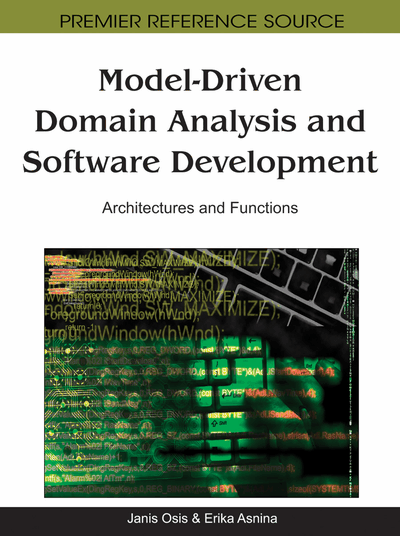# Topological Modeling for Model-Driven Domain Analysis and Software Development: Functions and Architectures

Janis Osis (Riga Technical University, Latvia) and Erika Asnina (Riga Technical University, Latvia)
Copyright: © 2011 |Pages: 25
DOI: 10.4018/978-1-61692-874-2.ch002
OnDemand PDF Download:
Available
\$37.50
No Current Special Offers

## Abstract

Model-driven software development has all chances to turn software development into software engineering. But this requires not only mature methodologies but also engineering models. An engineering model should satisfy five key characteristics, namely, abstraction, understandability, accuracy, predictiveness and inexpensiveness. This chapter discusses capabilities of a Topological Functioning Model (TFM) as such an engineering model for the purposes of domain analysis and software development in common. The TFM has functional and topological properties. The functional properties are cause-effect relations, cycle structure, inputs, and outputs. The topological properties are connectedness, closure, neighborhood, and continuous mapping. Thanks to its formal mathematical foundations, the TFM completely satisfies the mentioned characteristics of engineering models that is illustrated in the chapter.
Chapter Preview
Top

## Introduction

There are many ways how to describe semantics. In software development during the so called problem domain analysis mostly informal approaches and languages are used. There are several causes, and one of more important is that the problem domain itself is not well determined. Thus, developers explore the problem domain by parts, at the beginning trying to understand each fragment of the problem domain and only after that trying to join those fragments together in the holistic and more formal representation.

Indeed, the question about possibility of formal description of semantics still exists. The important property of diagrams used in software development is that they must provide very precise or even formal sense. In (Diskin, Kadish, Piessens, & Johnson, 2000) authors wrote that if some diagram D exists, then it has some sense M(D). This sense must be described in precise (it would be ideal, if mathematical) statements. This description makes some precise specification SD that has the precise semantic M(SD). Therefore, M(SD) is abstraction of formal intuitive sense M(D) and SD may be considered as some (internal) logic specification, that is hidden in the diagram D. They pointed that this described approach is typical (or desired) for diagrams used in software engineering.

There are many intuitive worlds that can provide sense M in the real world. The authors pointed out that in spite of that when one starts to think about M formal description, this huge amount is being narrowed until several domains of mathematical constructs, e.g. set theory, type theory, high-order predicate logic or category theory, etc. All these languages are universal and expressive. Therefore, any formal semantic (some specification SD) can be specified in any formal language of them.

But if we want to consider software development as an engineering discipline, then we need to take into account that not every formal language can be accepted for domain analysis by using models. Bran Selic (2003) enumerated five key characteristics that a useful and effective engineering model needs to satisfy. They are abstraction, understandability, accuracy, predictiveness and inexpensiveness (Selic, 2003):

• Abstraction is the most important characteristic. Usually, abstraction is the only available means of dealing with complex functionality and the structure of the system.

• Understandability makes the abstracted model expressive, reducing the amount of intellectual effort needed for model understanding.

• Accuracy makes the model useful. The model has to provide realistic representation of the modeled system features of interest.

• The model should be able to be used to predict the modeled system interesting implicit properties either through experimentation or through some formal analysis. In this case, the mathematical model is much better at predicting.

• The model should be inexpensive, so that it must be significantly cheaper in constructing and analysis than the modeled system.

Thus, an engineering model suitable for model-driven engineering is a formal mathematical model that supports abstraction and predictiveness, and at the same time it is understandable for all stakeholders (depending on the context), and cheap enough to be used for industrial tasks. As Unified Modeling Language (UML) developers’ and academic practice shows, one of such models could be Petri Nets and their derivations with some limitations in using. As our practice shows (and that what we demonstrate in this chapter), another one could be topological models of systems.

The chapter is organized as follows. The next section discusses advantages and weaknesses of Petri nets, and the background of topological models. Main properties and capabilities of the topological model, which support an engineering viewpoint on system functionality and structure, are described in section “Domain analysis with topological modeling”. The general framework of application of the topological model of system functioning for model-driven engineering is illustrated in section “Model-driven software development with topological modeling”.

## Complete Chapter List

Search this Book:
Reset